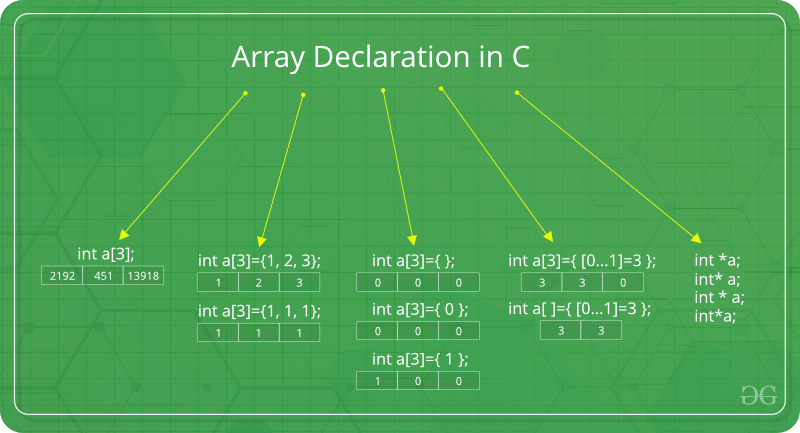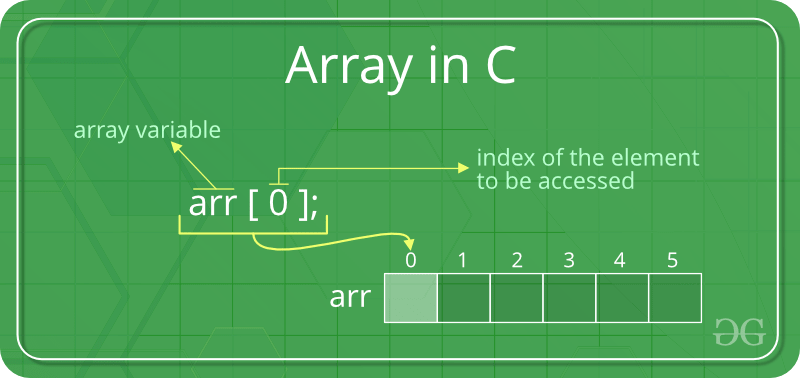# Arrays in C/C++

• Difficulty Level : Easy
• Last Updated : 14 Jun, 2022

Array

1. It is a group of variables of similar data type referred by single element.
2. It elements are stored in a continuous memory location.
3. The size of array should be mentioned while declaring it.
4. Array elements are always counted from zero (0) onward.
5. Array elements can be accessed using the position of the element in the array.
6. Array can have one or more dimensions.

An array in C/C++ or be it in any programming language is a collection of similar data items stored at contiguous memory locations and elements can be accessed randomly using indices of an array.  They can be used to store collection of primitive data types such as int, float, double, char, etc of any particular type. To add to it, an array in C/C++ can store derived data types such as the structures, pointers etc. Given below is the picture representation of an array.Why do we need arrays?
We can use normal variables (v1, v2, v3, ..) when we have a small number of objects, but if we want to store a large number of instances, it becomes difficult to manage them with normal variables. The idea of an array is to represent many instances in one variable.

• Code Optimization:  we can retrieve or sort the dataefficiently.
• Random access: We can get any data located at an index position.

• Size Limit: We can store only the fixed size of elements in the array. It doesn’t grow its size at runtime.

Array declaration in C/C++:Note: In above image int a={[0…1]=3}; this kind of declaration has been obsolete since GCC 2.5

There are various ways in which we can declare an array. It can be done by specifying its type and size, by initializing it or both.

Array declaration by specifying size

## C++

 `#include ` `using` `namespace` `std;`   `int` `main()` `{` `    ``// array declaration by specifying size` `    ``int` `arr1;` `  `  `    ``// With recent C/C++ versions, we can also` `    ``// declare an array of user specified size` `    ``int` `n = 10;` `    ``int` `arr2[n];` `    `  `    ``return` `0;` `}`   `// This code is contributed by sarajadhav12052009`

## C

 `#include `   `int` `main(``void``)` `{    ` `    ``// Array declaration by specifying size` `    ``int` `arr1;`   `    ``// With recent C/C++ versions, we can also` `    ``// declare an array of user specified size` `    ``int` `n = 10;` `    ``int` `arr2[n];` `    `  `    ``return` `(0);` `}`

Output

` `

Array declaration by initializing elements

## C++

 `// Array declaration by initializing elements` `#include ` `using` `namespace` `std; ` `int` `main()` `{` `    ``int` `arr[] = { 10, 20, 30, 40};` `    ``return` `0; ` `// Compiler creates an array of size 4.` `// above is same as  "int arr = {10, 20, 30, 40}"` `}`

## C

 `// Array declaration by initializing elements` `#include `   `int` `main(``void``)` `{` `    ``int` `arr[] = { 10, 20, 30, 40};` `    ``// Compiler creates an array of size 4.` `    ``// above is same as  "int arr = {10, 20, 30, 40}"` `  `  `    ``return` `(0);` `}`   `// This code is contributed by sarajadhav12052009`

Output

` `

Array declaration by specifying size and initializing elements

## C++

 `#include ` `using` `namespace` `std;`   `int` `main() ` `{` `    ``// Array declaration by specifying size and initializing` `    ``// elements` `    ``int` `arr = { 10, 20, 30, 40 };`   `    ``// Compiler creates an array of size 6, initializes first` `    ``// 4 elements as specified by user and rest two elements as` `    ``// 0. above is same as  "int arr[] = {10, 20, 30, 40, 0, 0}"` `    `  `    ``return` `0;` `}`   `// This code is contributed by sarajadhav12052009`

## C

 `#include `   `int` `main(``void``)` `{` `    ``// Array declaration by specifying size and initializing` `    ``// elements` `    ``int` `arr = { 10, 20, 30, 40 };`   `    ``// Compiler creates an array of size 6, initializes first` `    ``// 4 elements as specified by user and rest two elements as` `    ``// 0. above is same as  "int arr[] = {10, 20, 30, 40, 0, 0}"` `    `  `    ``return` `(0);` `}`

Advantages of an Array in C/C++:

1. Random access of elements using array index.
2. Use of fewer line of code as it creates a single array of multiple elements.
4. Traversal through the array becomes easy using a single loop.
5. Sorting becomes easy as it can be accomplished by writing fewer line of code.

Disadvantages of an Array in C/C++:

1. Allows a fixed number of elements to be entered which is decided at the time of declaration. Unlike a linked list, an array in C is not dynamic.
2. Insertion and deletion of elements can be costly since the elements are needed to be managed in accordance with the new memory allocation.

• Accessing Array Elements:
Array elements are accessed by using an integer index. Array index starts with 0 and goes till size of array minus 1.
• Name of the array is also a pointer to the first element of array.Example:

## C

 `#include `   `int` `main()` `{` `    ``int` `arr;` `    ``arr = 5;` `    ``arr = -10;` `    ``arr[3 / 2] = 2; ``// this is same as arr = 2` `    ``arr = arr;`   `    ``printf``(``"%d %d %d %d"``, arr, ` `           ``arr, arr, arr);`   `    ``return` `0;` `}`

## C++

 `#include ` `using` `namespace` `std;`   `int` `main()` `{` `    ``int` `arr;` `    ``arr = 5;` `    ``arr = -10;`   `    ``// this is same as arr = 2` `    ``arr[3 / 2] = 2;` `    ``arr = arr;`   `    ``cout << arr << ``" "` `<< arr << ``" "` `<< arr << ``" "` `         ``<< arr;`   `    ``return` `0;` `}`

Output

`5 2 -10 5`

No Index Out of bound Checking:
There is no index out of bounds checking in C/C++, for example, the following program compiles fine but may produce unexpected output when run.

## C

 `// This C program compiles fine` `// as index out of bound` `// is not checked in C.`   `#include `   `int` `main()` `{` `    ``int` `arr;`   `    ``printf``(``"%d "``, arr);` `    ``printf``(``"%d "``, arr[-2]);`   `    ``return` `0;` `}`

## C++

 `// This C++ program compiles fine` `// as index out of bound` `// is not checked in C.`   `#include ` `using` `namespace` `std;`   `int` `main()` `{` `    ``int` `arr;`   `    ``cout << arr << ``" "``;` `    ``cout << arr[-2] << ``" "``;`   `    ``return` `0;` `}`

Output

`211343841 4195777 `

In C, it is not a compiler error to initialize an array with more elements than the specified size. For example, the below program compiles fine and shows just Warning.

## C

 `#include ` `int` `main()` `{`   `    ``// Array declaration by initializing it ` `    ``// with more elements than specified size.` `    ``int` `arr = { 10, 20, 30, 40, 50 };`   `    ``return` `0;` `}`

Warnings:

```prog.c: In function 'main':
prog.c:7:25: warning: excess elements in array initializer
int arr = { 10, 20, 30, 40, 50 };
^
prog.c:7:25: note: (near initialization for 'arr')
prog.c:7:29: warning: excess elements in array initializer
int arr = { 10, 20, 30, 40, 50 };
^
prog.c:7:29: note: (near initialization for 'arr')
prog.c:7:33: warning: excess elements in array initializer
int arr = { 10, 20, 30, 40, 50 };
^
prog.c:7:33: note: (near initialization for 'arr')```
• Note: The program won’t compile in C++. If we save the above program as a .cpp, the program generates compiler error “error: too many initializers for ‘int '”

The elements are stored at contiguous memory locations
Example:

## C

 `// C program to demonstrate that` `// array elements are stored` `// contiguous locations`   `#include ` `int` `main()` `{` `    ``// an array of 10 integers.  ` `    ``// If arr is stored at` `    ``// address x, then arr is` `    ``// stored at x + sizeof(int)` `    ``// arr is stored at x + ` `    ``// sizeof(int) + sizeof(int)` `    ``// and so on.` `    ``int` `arr, i;`   `    ``printf``(``"Size of integer in this compiler is %lu\n"``,` `           ``sizeof``(``int``));`   `    ``for` `(i = 0; i < 5; i++)` `        ``// The use of '&' before a variable name, yields` `        ``// address of variable.` `        ``printf``(``"Address arr[%d] is %p\n"``, i, &arr[i]);`   `    ``return` `0;` `}`

## C++

 `// C++ program to demonstrate that array elements` `// are stored contiguous locations`   `#include ` `using` `namespace` `std;`   `int` `main()` `{` `    ``// an array of 10 integers.  ` `    ``// If arr is stored at` `    ``// address x, then arr is ` `    ``// stored at x + sizeof(int)` `    ``// arr is stored at x + ` `    ``// sizeof(int) + sizeof(int)` `    ``// and so on.` `    ``int` `arr, i;`   `    ``cout << ``"Size of integer in this compiler is "` `         ``<< ``sizeof``(``int``) << ``"\n"``;`   `    ``for` `(i = 0; i < 5; i++)` `        ``// The use of '&' before a variable name, yields` `        ``// address of variable.` `        ``cout << ``"Address arr["` `<< i << ``"] is "` `<< &arr[i]` `             ``<< ``"\n"``;`   `    ``return` `0;` `}`

Output

```Size of integer in this compiler is 4

Another way to traverse the array

## C++

 `#include` `using` `namespace` `std;`   `int` `main()` `{` `    ``int` `arr={11,12,13,14,15,16};` `    ``// Way 1` `    ``for``(``int` `i=0;i<6;i++)` `        ``cout<

## C

 `#include`   `int` `main(``void``)` `{` `    ``int` `arr = {11, 12, 13, 14, 15, 16};` `  `  `    ``// Way 1` `    ``for``(``int` `i = 0; i < 6; i++)` `          ``printf``(``"%i "``, arr[i]);`   `    ``printf``(``"\n"``);` `  `  `      ``// Way 2` `    ``printf``(``"By Other Method: \n"``);` `  `  `    ``for``(``int` `i = 0; i < 6; i++)` `          ``printf``(``"%i "``, i[arr]);`   `    ``printf``(``"\n"``);`   `    ``return` `(0);` `}`   `// This code is contributed by sarajadhav12052009`

Output

```11 12 13 14 15 16
By Other Method:
11 12 13 14 15 16 ```

Array vs Pointers
Arrays and pointers are two different things (we can check by applying sizeof). The confusion happens because array name indicates the address of first element and arrays are always passed as pointers (even if we use square bracket). Please see Difference between pointer and array in C? for more details.
What is vector in C++?
A vector in C++ is a class in STL that represents an array. The advantages of vector over normal arrays are,

• We do not need pass size as an extra parameter when we declare a vector i.e, Vectors support dynamic sizes (we do not have to initially specify size of a vector). We can also resize a vector.
• Vectors have many in-built functions like, removing an element, etc.

To know more about functionalities provided by vector, please refer vector in C++ for more details.